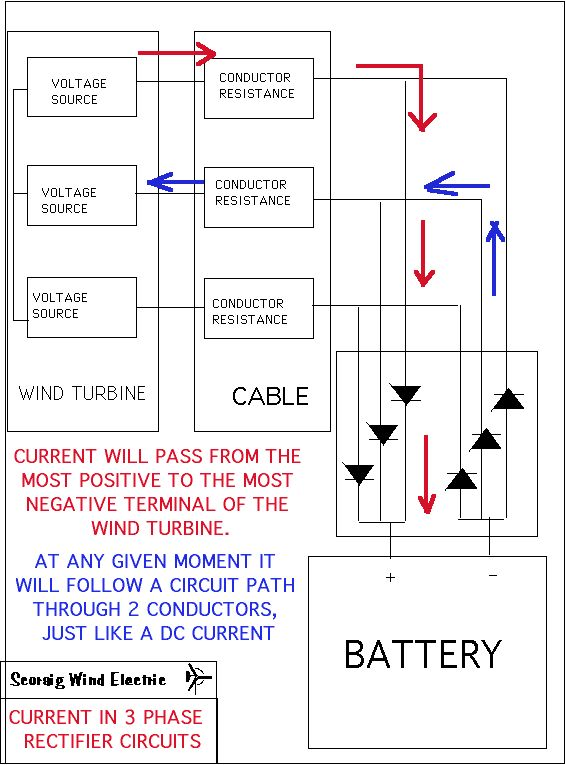# Wiring loss in 3 phase wind systems.

There is some confusion about how to calculate losses in the cables of a 3 phase wind system.  If the 3 wires are feeding a rectifier, charging a battery, then the current in the cables is dominated by the need to supply DC at battery voltage to the load.

The cables may change, but the current tends to remain constant.Paul Gipe's question
What is the power lost in conductors from a 850 Watt permanent-magnet,
three-phase alternator feeding a diode bridge rectifier delivering a
nominal 24 VDC to a battery bank. The rpm, voltage, and current of the
three-phase alternator varies with wind speed. There are three cables
(conductors) between the wind turbine's alternator and the diode bridge.
There are two conductors from the diode bridge to the batteries. The
conductors are #8 AWG with an AC resistance of 0.78 Ohms/1000 feet. There
is 150 feet from the wind turbine to the diode bridge, and an insignificant
distance from the diode bridge to the batteries.

The DC current is found by dividing watts by volts.  this gives 850/24 = 35 amps.

If you want to analyse situtation mathematically, current in each conductor is 35 amps for 2/3 of the time.  The rms current in each conductor is therefore (2/3)^.5=0.82 times 35 amps = 29 amps (rms).  Resistance of each conductor is 0.78 times the cable run of 150/1000 feet,  giving  0.117 ohms.
Power loss is I^2*0.117
which is 2/3*35^2*0.117
which is 1/3*35^2*.234 for each conductor
which is 35^2*.234=287W in total.

This is a 34% loss!

An easier way to analyse the situation (which also give the same answer) is to say that at any given instant the DC current 35 amps is flowing around a circuit path with resistance equal to 0.78*(cable run in feet/1000).  Cable run is 300 feet for the full circuit.

Finally I should point out that the above is strictly only true if the internal loss is small.  As loss increases, the situation becomes much more complex, since more than 2 wires will start to conduct at once during the changeover.  But the above answer will be accurate enough for practical purposes, given that we are arguing about such large differences in our answers.

Hugh

October 1999